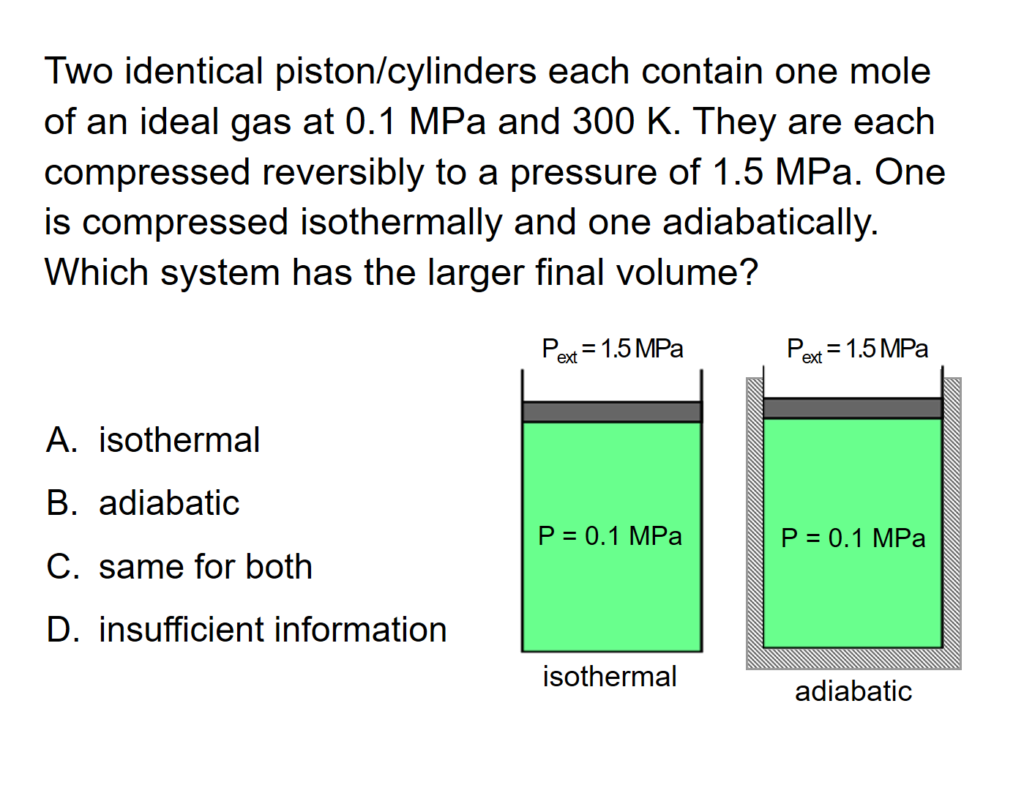LearnChemE

#### First Law - Closed Systems: ConcepTest and Example Problem

Try to answer this ConcepTest and solve the example problem before using this module. Studies show that trying to answer the questions before studying material improves learning and retention. We suggest that you write down the reasons for your answers. By the end of this module, you should be able to answer these on your own. Answers will be given later in this module.##### Example Problem 1

Calculate the final conditions for the adiabatic reversible compression of an ideal gas that compresses to 1 cm3. The initial conditions are:  V1 = 10 cm3,   P1 = 1 bar,   T1 = 300